Courses

# Test: Reactions Of Alkene

## 14 Questions MCQ Test Chemistry for JEE | Test: Reactions Of Alkene

Description
This mock test of Test: Reactions Of Alkene for JEE helps you for every JEE entrance exam. This contains 14 Multiple Choice Questions for JEE Test: Reactions Of Alkene (mcq) to study with solutions a complete question bank. The solved questions answers in this Test: Reactions Of Alkene quiz give you a good mix of easy questions and tough questions. JEE students definitely take this Test: Reactions Of Alkene exercise for a better result in the exam. You can find other Test: Reactions Of Alkene extra questions, long questions & short questions for JEE on EduRev as well by searching above.
QUESTION: 1

Solution:
QUESTION: 2

Solution:
QUESTION: 3

### What is major product in the following reaction?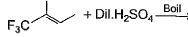Solution:
QUESTION: 4

What is formed predominantly in the reaction?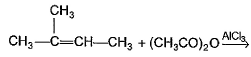Solution:
QUESTION: 5

What is the major addition product in the reaction given below?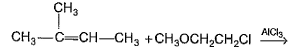Solution:
QUESTION: 6

Consider the following reaction.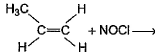The major product is

Solution:
QUESTION: 7

What is major product in the reaction?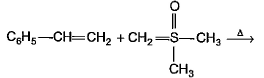Solution:
*Multiple options can be correct
QUESTION: 8

Direction (Q. Nos. 8 -11) This section contains 4 multiple choice questions. Each question has four choices (a), (b), (c) and (d), out of which ONE or MORE THAN ONE are correct.

Q. What is /are the expected product in the reaction ?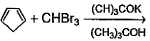Solution:
*Multiple options can be correct
QUESTION: 9

What are the expected product(s) in the following reaction?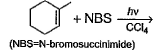Solution:

The correct answer is Option A,B,C,D.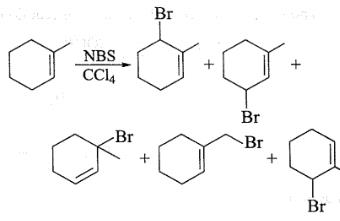*Multiple options can be correct
QUESTION: 10

What products are expected in the following reaction?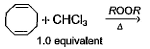Solution:
*Multiple options can be correct
QUESTION: 11

In which of the following reaction, reactant(s) and product(s) are correctly matched?

Solution:
QUESTION: 12

Direction (Q. Nos. 12 and13) This section contains a paragraph, describing theory, experiments, data, etc. Two questions related to the paragraph have been given. Each question has only one correct answer among the four given options (a), (b), (c) and (d).

Passage

Caryophyllene (C15H24) contain a six membered ring and on ozonolysis gives following product.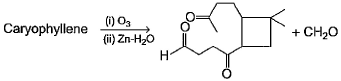Q. The structure of caryophyllene is

Solution:
QUESTION: 13

Caryophyllene (C15H24) contain a six membered ring and on ozonolysis gives following product.Q. If caryophyllene is treated with 1.0 mole of HCI, a ring closure reaction takes place to form monochloride. What is the most likely product of this reaction?

Solution:
QUESTION: 14

Direction (Q . No. 14) Choices for the correct combination of elements from Column I and Column II are given as options (a), (b), (c) and (d), out of which one is correct.

Q. Match the alkenes from Column I with the stereochemistry of addition product(s) obtained with Br2/CCI4 in presence of FeBr3.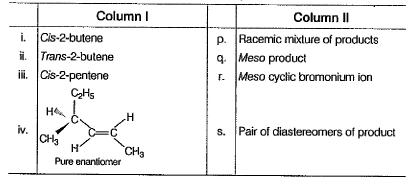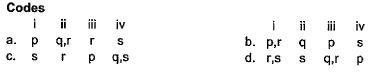Solution: